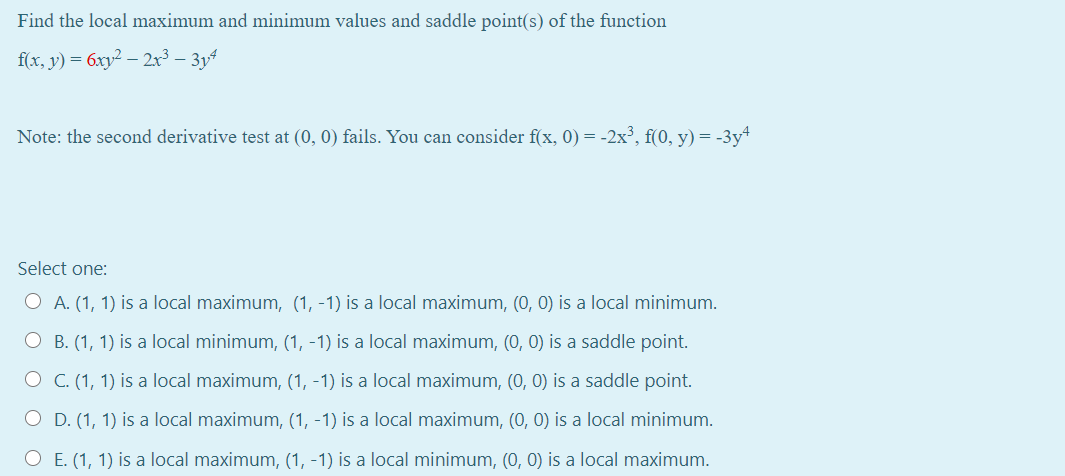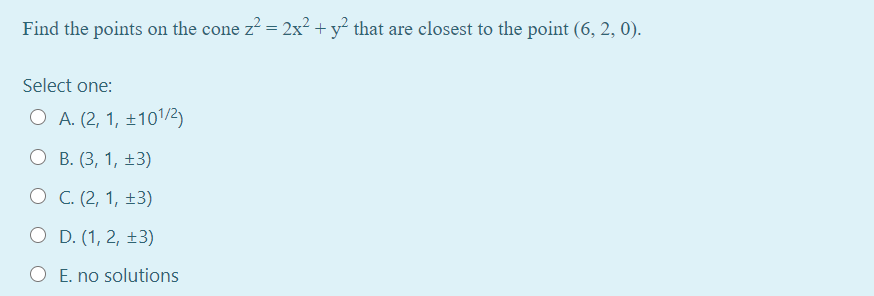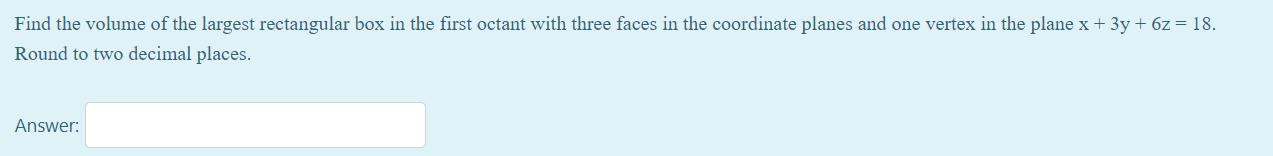# Question Solved1 AnswerFind the local maximum and minimum values and saddle point(s) of the function f(x, y) = 6xy2 – 2x3 – 3y4 Note: the second derivative test at (0, 0) fails. You can consider f(x, 0) = -2x), f(0, y) = -3y4 Select one: O A. (1, 1) is a local maximum, (1, -1) is a local maximum, (0, 0) is a local minimum. O B. (1, 1) is a local minimum, (1, -1) is a local maximum, (0, 0) is a saddle point. O C. (1, 1) is a local maximum, (1, -1) is a local maximum, (0, 0) is a saddle point. O D. (1, 1) is a local maximum, (1, -1) is a local maximum, (0, 0) is a local minimum. O E. (1, 1) is a local maximum, (1, -1) is a local minimum, (0, 0) is a local maximum. Find the points on the cone z? = 2x2 + y2 that are closest to the point (6,2,0). Select one: O A. (2, 1, 101/2) O B.(3, 1, +3) O C. (2, 1, +3) O D. (1, 2, +3) O E. no solutions Find the volume of the largest rectangular box in the first octant with three faces in the coordinate planes and one vertex in the plane x + 3y + 6z = 18. Round to two decimal places. Answer:Transcribed Image Text: Find the local maximum and minimum values and saddle point(s) of the function f(x, y) = 6xy2 – 2x3 – 3y4 Note: the second derivative test at (0, 0) fails. You can consider f(x, 0) = -2x), f(0, y) = -3y4 Select one: O A. (1, 1) is a local maximum, (1, -1) is a local maximum, (0, 0) is a local minimum. O B. (1, 1) is a local minimum, (1, -1) is a local maximum, (0, 0) is a saddle point. O C. (1, 1) is a local maximum, (1, -1) is a local maximum, (0, 0) is a saddle point. O D. (1, 1) is a local maximum, (1, -1) is a local maximum, (0, 0) is a local minimum. O E. (1, 1) is a local maximum, (1, -1) is a local minimum, (0, 0) is a local maximum. Find the points on the cone z? = 2x2 + y2 that are closest to the point (6,2,0). Select one: O A. (2, 1, 101/2) O B.(3, 1, +3) O C. (2, 1, +3) O D. (1, 2, +3) O E. no solutions Find the volume of the largest rectangular box in the first octant with three faces in the coordinate planes and one vertex in the plane x + 3y + 6z = 18. Round to two decimal places. Answer:
More
Transcribed Image Text: Find the local maximum and minimum values and saddle point(s) of the function f(x, y) = 6xy2 – 2x3 – 3y4 Note: the second derivative test at (0, 0) fails. You can consider f(x, 0) = -2x), f(0, y) = -3y4 Select one: O A. (1, 1) is a local maximum, (1, -1) is a local maximum, (0, 0) is a local minimum. O B. (1, 1) is a local minimum, (1, -1) is a local maximum, (0, 0) is a saddle point. O C. (1, 1) is a local maximum, (1, -1) is a local maximum, (0, 0) is a saddle point. O D. (1, 1) is a local maximum, (1, -1) is a local maximum, (0, 0) is a local minimum. O E. (1, 1) is a local maximum, (1, -1) is a local minimum, (0, 0) is a local maximum. Find the points on the cone z? = 2x2 + y2 that are closest to the point (6,2,0). Select one: O A. (2, 1, 101/2) O B.(3, 1, +3) O C. (2, 1, +3) O D. (1, 2, +3) O E. no solutions Find the volume of the largest rectangular box in the first octant with three faces in the coordinate planes and one vertex in the plane x + 3y + 6z = 18. Round to two decimal places. Answer: